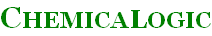Formulations Description Scientific Formulation(IAPWS-95) The scientific formulation is described in the following reference: Release on the IAPWS Formulation 1995 for the Thermodynamic Properties of Ordinary Water Substance for General and Scientific Use, The International Association for the Properties of Water and Steam, Frederica, Denmark, Sept. 1996.The scientific formulation is valid in the entire stable fluid region of water from the melting pressure curve starting at 251.165 K to 1273 K at pressures up to 1,000 MPa. The formulation can also be extrapolated to pressures up to about 100 GPa and temperatures up to 5000 K.SteamTab Quad imposes the following temperature and pressure restrictions:```Pressure (P, bar): 0 <= P <= 100,000 Temperature (T, K): 190 <= T <= 5000``` Industrial Formulation(IAPWS-IF97) The industrial formulation is described in the following reference: Release on the IAPWS Industrial Formulation 1997 for the Thermodynamic Properties of Water and Steam, The International Association for the Properties of Water and Steam, Erlangen, Germany, 1997.This formulation has the following range of applicability:```Pressure (P, bar): 0 <= P <= 1,000 Temperature (T, K): 273.15 <= T <= 1,073.15 (for P <= 1,000) 1073.15 <= T <= 2,273.17 (for P <= 100)``` Old Scientific Formulation(NBS/NRC-84) The NBS/NRC formulation is described in the following reference: NBS/NRC Steam Tables, L. Haar, J. S. Gallagher and G. S. Hell. Hemisphere Publishing Corporation, 1984. Generally, the NBS/NRC formulation has the following range of applicability:```Pressure (P, bar): 0 <= P <= 30,000 Temperature (T, K): 260 <= T <= 2,500``` Old Industrial Formulation(IFC-67) The IFC 1967 formulation is described in the following reference: The 1967 IFC Formulation for Industrial Use, ASME Steam Tables, Sixth Edition, ASME Press. Generally, the IFC 1967 formulation has the following range of applicability:```Pressure (P, bar): 0 <= P <= 1,000 Temperature (T, K): 273.16 <= T <= 1,073.15```